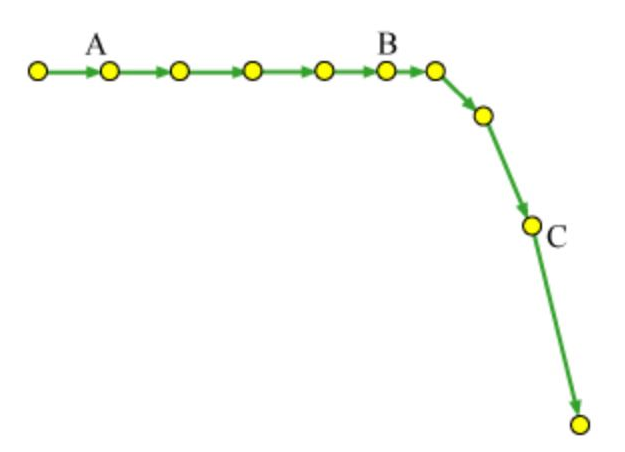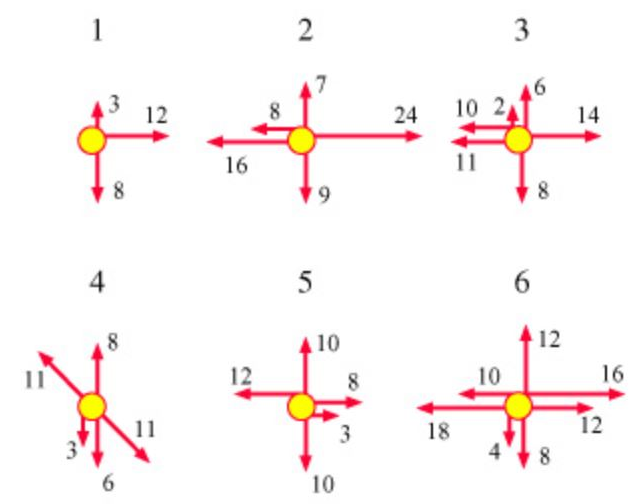# Problem: The next four questions are related to the force diagrams numbered 1 to 6. (Figure 2) These diagrams represent the forces acting on a moving object. The number next to each arrow represents the magnitude of the force in newtons.Which of these diagrams may possibly correspond to the situation at point A on the motion diagram?

###### FREE Expert Solution

The object is moving at a constant velocity at point A.

89% (459 ratings)###### Problem Details

The next four questions are related to the force diagrams numbered 1 to 6. (Figure 2) These diagrams represent the forces acting on a moving object. The number next to each arrow represents the magnitude of the force in newtons.Which of these diagrams may possibly correspond to the situation at point A on the motion diagram?Frequently Asked Questions

What scientific concept do you need to know in order to solve this problem?

Our tutors have indicated that to solve this problem you will need to apply the Forces & Kinematics concept. You can view video lessons to learn Forces & Kinematics. Or if you need more Forces & Kinematics practice, you can also practice Forces & Kinematics practice problems.

What professor is this problem relevant for?

Based on our data, we think this problem is relevant for Professor Koutroulakis' class at UCSB.